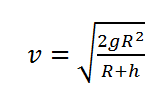# Escape Velocity calculator

This CalcTown calculator calculates the escape velocity of an object from Earth, given the height it is projected from.

km

#### Result

Km/swhere

R is the Radius of the Earth=6400 Km

h is the height from which the object is to be projected

g is the value of acceleration due to gravity on Earth's surface=9.80 m/s2